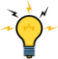# A Logarithmic Slider ControlHomeBack To Tips Page

Here is an MFC implementation of a logarithmic slider control. It is subclassed from CSliderCtrl. Note that in addition to the logarithmic/floating point interface, there is a Linear Position interface, which is sometimes useful.

Here are samples from the code

```void CLogSlider::SetRange(double L, double H, UINT resolution)
{
ASSERT(L < H);
low = L;
high = H;
CSliderCtrl::SetRange(0, (int)resolution);
} // CLogSlider::SetRange```

This function sets the slider range from one bound to another. These usually differ by several orders of magnitude, e.g.,

`c_MySlider.SetRange(1e-4, 1e4, 1000);`

The third parameter is the resolution, that is, how many points appear between the lower and upper bound. In this case, the lower to upper bound is a factor of 1e8, or 10,000,000. The resolution is 1000, so you have 1000 increments in the range of 10-4..104.

```BOOL CLogSlider::SetPos(double pos)
{
if(pos < low)
return FALSE;
if(pos > high)
return FALSE;

int L;
int H;
CSliderCtrl::GetRange(L, H);

double loglow = log10(low);
double loghigh = log10(high);

double range = loghigh - loglow;

double ratio = range / (double)(H - L);

double logpos = log10(pos);

double logoffset = logpos - loglow;

double linear = logoffset / ratio;

int newpos = (int)(linear + 0.5);
if(newpos < 0)
newpos = 0; // roundoff issues may create this

CSliderCtrl::SetPos( newpos );

return TRUE;
} // CLogSlider::SetPos```

This function takes a floating point number which represents a position and sets the control to approximately that position. It is important to recognize that having done this, a GetPos will not necessarily return the same value that was used for SetPos, because of the loss in precision. The resolution parameter of SetRange can be used to get greater accuracy, but ultimately you are limited by the pixel resolution of your display (you can really only move it the control in integral pixel units).

```double CLogSlider::GetPos()
{
int L;
int H;
CSliderCtrl::GetRange(L, H);

double loglow = log10(low);
double loghigh = log10(high);

double range = loghigh - loglow;

double ratio = range / (double)(H - L);

int linearpos = CSliderCtrl::GetPos(); // linear position

double pos = (double)linearpos * ratio;

double adjustedpos = loglow + pos;
return result;
} // CLogSlider::GetPos```

This takes the linear position stored in the control and produces a logarithmic result. See the caveat in the previous paragraph discussing SetPos: the position returned will almost certainly not be the same floating-point value passed in by SetPos, because of the loss of precision given the resolution.### Notes:

I do not have time right now to write a code example. This was taken directly from working code and copied verbatim, so I have every expectation it works. If I have time to write an example, I will update this page.The views expressed in these essays are those of the author, and in no way represent, nor are they endorsed by, Microsoft.